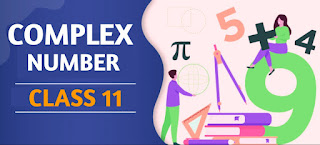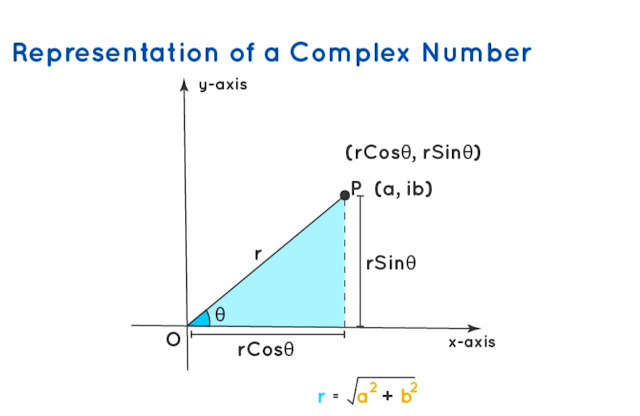# Complex Numbers Class 11 Mathematics Solutions | Exercise - 7.2## Chapter - 7  Complex Numbers

In class 11 mathematics, we learn about complex number which is a number system that extends the real numbers with a specific element denoted i, called the imaginary unit and satisfying the equation i² = -1. Complex number can be expressed in the form a + ib, where a and b are real numbers. In any complex number a+ib, a is called the real part, and b is called the imaginary part.

Later in this chapter we are going to learn the concept of complex numbers and their properties. In addition that, we will also learn the basic operations like addition, subtraction, multiplication, and division of complex numbers. Before, we move forward it is better to learn some terms and important formulas related to complex number. So let's have a brief introduction of the complex number chapter.

### Introduction

Earlier in the text we discussed about the set of real numbers. We have also seen how real numbers can be represented by points in a straight line, called the real line or the number line. Every real number corresponds to a point in the real line and conversely. One important fact about real number is

"The square of a real number is never negative."

A problem occurs when we solve the equation of the type x² + 4 = 0 Because there is no value of x in the real number system that the square of x is negative. In order to overcome this difficulty, we have to extend the system of numbers. This is done by introducing new set of numbers called complex numbers. It is believed that Cardon used complex numbers in 1546.

### Conjugate of a Complex Number

Let z = a + ib be a complex number. Then the conjugate of the complex number z denoted by ˉz = a - ib =(a - b) Similarly if z = a - ib be the given complex number, then its conjugate ˉz is given by ˉz = a + ib.

### Absolute Value of a Complex Number

The absolute value of a complex number z = a + ib is defined as the non-negative real number (a² + b²)½. It is denoted by |z| or |a + ib |. The absolute value of a complex number is also known as the modulus of the complex number.

### Powers of i

We have defined i as that number whose square is -1, and it is the imaginary unit. Any real multiple of i is an imaginary number. Since,
i² = - 1 we have
i³ = i²×i = (- 1)× i = - i
i⁴ = (i²)² = (- 1)² = 1
i⁵ = (i⁴)× i = (1)× i = i
i⁶ = (i²)³ = (- 1)³ = - 1
i¹⁰ = (i²)⁵ = (- 1)⁵ = - 1### Exercise - 7.2

In this PDF, you'll only find the solution of class 11 complex number chapter. It contains all the solutions of exercise- 7.2. If you want the solutions of other exercises then you'll find them above this PDF. Just click on the button and you'll reach your destination.

NoteScroll the PDF to view all Solution

You are not allowed to post this PDF in any website or social platform without permission.

## Is Class 11 Mathematics Guide Helpful For Student ?

I have published this Notes for helping students who can't solve difficult maths problems. Student should not fully depend on this note for completing all the exercises. If you totally depend on this note and simply copy as it is then it may affect your study.

Student should also use their own will power and try to solve problems themselves. You can use this mathematics guide PDF as a reference. You should check all the answers before copying because all the answers may not be correct. There may be some minor mistakes in the note, please consider those mistakes.

## How to secure good marks in Mathematics ?

As, you may know I'm also a student. Being a student is not so easy. You have to study different subjects simultaneously. From my point of view most of the student are weak in mathematics. You can take me as an example, I am also weak in mathematics. I also face problems while solving mathematics questions.

If you want to secure good marks in mathematics then you should practise them everyday. You should once revise all the exercise which are already taught in class. When you are solving maths problems, start from easy questions that you know already. If you do so then you won't get bored.

Maths is not only about practising, especially in grade 11 you to have the basic concept of the problem. When you get the main concept of the problem then you can easily any problems in which similar concept are applied.

When your teacher tries to make the concept clear by giving examples then all students tries to remember the same example but you should never do that. You can create your own formula which you won't forget later.

If you give proper time for your practise with proper technique then you can definitely score a good marks in your examination.

Disclaimer: This website is made for educational purposes. If you find any content that belongs to you then contact us through the contact form. We will remove that content from our as soon as possible.

If you have any queries then feel free to comment down.

No Comment